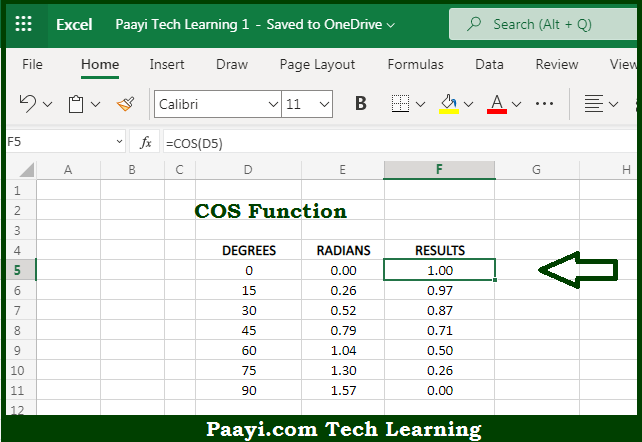# Learn How to Use Microsoft Excel COS Function

Written by | 0 Comments | 622 Views

In this article, you will learn how to use the Microsoft Excel COS function and its prime function in Microsoft Excel. You will also get to know the Microsoft Excel COS function return value and syntax with the help of some examples.

Microsoft Excel COS Function

The main purpose of the Microsoft Excel COS function is to get the cosine of the angle provided in radians. That implies, with the help of the COS function you can able to return the cosine of an angle provided in the radians. It should be noted that you can supply an angle to COS in degrees, and you can convert it t radians using the RADIANS function. So, with the help of the COS function, you can able to get the cosine of an angle provided in the radians.

Return Value of COS Function

The return value will be the cosine of an angle.

Syntax of COS Function

=COS(number)

Where the arguments:

• number: This is the angle at which you want to get the cosine value.

## How to Use Microsoft Excel COS Function?So we know that Microsoft Excel COS function you can able to get the cosine of the angle provided in radians. That implies, with the help of the COS function you can able to return the cosine of an angle provided in the radians. It should be noted that you can supply an angle to COS in degrees, and you can convert it t radians using the RADIANS function. So, with the help of the COS function, you can able to get the cosine of an angle provided in the radians.